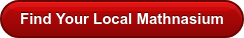Number Sense Blog

By Mathnasium | Added Sep 23, 2019Today is the Autumnal Equinox here in the Northern Hemisphere! Sometimes considered the beginning of fall, the autumnal equinox is actually one of two times a year when the length of day and night are equal. We thought this would be a perfect time to talk about the meaning and importance of the equal sign (=).

In math, symbols convey very specific meanings, and a proper understanding of these meanings is essential to a true understanding of math. As math tutors, we know how important it is for students to have a correct and comfortable understanding of these symbols and their meanings.

Younger students sometimes think the equal sign means “the answer is.” While this can be accurate for some mathematical phrases, for example, 1 + 3 = 4 (one plus three, the answer is four), it doesn’t hold up when you begin using slightly more advanced phrasing such as 1 + 3 = 2 + 2 (one plus three, the answer is two plus two).

The meaning of the equal sign (=) according to the Mathnasium Math Dictionary™ is “being the same in value as.” If students think of the equal sign (=) in this way, then mathematical phrasing of any kind becomes much clearer.

1 + 3 = 4 (One plus three is the same value as four.)

1 + 3 = 2 + 2 (One plus three is the same value as two plus two.)

A = L x W (The area of a rectangle is the same value as its length times its width.)A correct understanding of the meaning of the equal sign is critical to Number Sense, performance in math classes at all levels and, according to researchers from Texas A&M University, to achievement in career fields that require any kind of mathematical skills.

As adults, we sometimes forget how easy it is for children to misunderstand the meanings of the mathematical symbols we take for granted. When teachers or local math tutors spend just a little bit of time ensuring that students have a solid understanding of these symbols, the effects can reach far into their academic and professional futures.

Happy Fall Equinox from all of us here at Mathnasium, where today ⁠— and every day ⁠— we’re changing lives through math!Favorites

Bedtime Math: http://bedtimemath.org/

National PTA: http://www.pta.org/

NCTM: http://www.nctm.org/

Numberphile: http://www.numberphile.com/

PTO Today: http://www.ptotoday.com/

STEM Connector: http://blog.stemconnector.org/

Before I came to Mathnasium, I could sum up everything I felt about math in one word: 'EVIL!' I hated math. ... Mathnasium has been my safe haven. They truly have shown me the light when it came to addressing my fear and provided me with the tools that I need to rebuild my prior knowledge so that I won't forget it. Math is no longer a subject I shy away from but it is a subject I can boldly accept and understand.